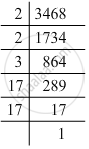Advertisement Remove all ads

# By What Number Should Each of the Following Numbers Be Multiplied to Get a Perfect Square ? Also, Find the Number Whose Square is the New Number. 3468 - Mathematics

By what number should each of the following numbers be multiplied to get a perfect square ? Also, find the number whose square is the new number.

3468

Advertisement Remove all ads

#### Solution

3468 = 2 x 2 x 3 x 17 x 17Grouping them into pairs of equal factors:
3468 = (2 x 2) x (17 x 17) x 3
The factor 3 is not paired. For a number to be a perfect square, each prime factor has to be paired. Hence, 3468 must be multiplied by 3 for it to be a perfect square.
The new number would be (2 x 2) x (17 x 17) x (3 x 3).
Furthermore, we have:
(2 x 2) x (17 x 17) x (3 x 3) = (2 x 3 x 17) x (2 x 3 x 17)
Hence, the number whose square is the new number is:
2 x 3 x 17 = 102

Is there an error in this question or solution?
Advertisement Remove all ads

#### APPEARS IN

RD Sharma Class 8 Maths
Chapter 3 Squares and Square Roots
Exercise 3.1 | Q 7.6 | Page 4
Advertisement Remove all ads

#### Video TutorialsVIEW ALL 

Advertisement Remove all ads
Share
Notifications

View all notifications

Forgot password?
Course